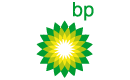# 10 Large Cap High Yields With Single P/E Ratios

+5.34%
Upside
31.28
Market
32.95
TrefisBP
BP

This article was submitted by Dividend Yield using our Trefis Contributors tool

High yield dividend stocks are a great opportunity for dividend investors. Especially in the case of higher capitalized companies, the possibility for high dividend payments in the long-run is much higher. They are big and generate huge cash flows. However, I screened all stocks with a market capitalization over USD 10 billion with a dividend yield of more than 5 percent. In order to exclude stocks with a higher valuation, I decided to select only those stocks with a single P/E ratio. As result, ten companies remained of which four stocks have a double digit yield. Some of the companies, like Telefonica (TEF), have announced to reduce the upcoming dividends. Six of the results have a buy or better rating.

Here are my favorite stocks:

Relevant Articles

1. YPF SA (YPF) has a market capitalization of \$11.31 billion. The company employs 13,370 people, generates revenues of \$10,194.60 million and has a net income of \$1,336.60 million. The firm’s earnings before interest, taxes, depreciation and amortization (EBITDA) amounts to \$3,404.51 million. Because of these figures, the EBITDA margin is 33.40 percent (operating margin 21.46 percent and the net profit margin finally 13.11 percent).

The total debt representing 16.72 percent of the company’s assets and the total debt in relation to the equity amounts to 40.91 percent. Due to the financial situation, a return on equity of 25.51 percent was realized. Twelve trailing months earnings per share reached a value of \$3.36. Last fiscal year, the company paid \$2.61 in form of dividends to shareholders.

Here are the price ratios of the company: The P/E ratio is 8.57, P/S ratio 1.27 and P/B ratio 2.57. Dividend Yield: 11.80 percent. The beta ratio is 0.72.

2. TOTAL S.A. (TOT) has a market capitalization of \$126.78 billion. The company employs 92,855 people, generates revenues of \$219,862.20 million and has a net income of \$16,608.14 million. The firm’s earnings before interest, taxes, depreciation and amortization (EBITDA) amounts to \$41,865.56 million. Because of these figures, the EBITDA margin is 19.04 percent (operating margin 15.00 percent and the net profit margin finally 7.55 percent).

The total debt representing 19.65 percent of the company’s assets and the total debt in relation to the equity amounts to 47.37 percent. Due to the financial situation, a return on equity of 19.11 percent was realized. Twelve trailing months earnings per share reached a value of \$7.18. Last fiscal year, the company paid \$3.01 in form of dividends to shareholders.

Here are the price ratios of the company: The P/E ratio is 7.83, P/S ratio 0.61 and P/B ratio 1.41. Dividend Yield: 6.88 percent. The beta ratio is 1.01.

3. Eni S.p.A. (E) has a market capitalization of \$83.57 billion. The company employs 79,641 people, generates revenues of \$145,899.80 million and has a net income of \$10,341.64 million. The firm’s earnings before interest, taxes, depreciation and amortization (EBITDA) amounts to \$34,036.07 million. Because of these figures, the EBITDA margin is 23.33 percent (operating margin 15.82 percent and the net profit margin finally 7.09 percent).

The total debt representing 20.67 percent of the company’s assets and the total debt in relation to the equity amounts to 53.30 percent. Due to the financial situation, a return on equity of 12.91 percent was realized. Twelve trailing months earnings per share reached a value of \$5.02. Last fiscal year, the company paid \$2.75 in form of dividends to shareholders.

Here are the price ratios of the company: The P/E ratio is 9.19, P/S ratio 0.64 and P/B ratio 1.14. Dividend Yield: 6.08 percent. The beta ratio is 1.07.

Take a closer look at the full table of high yield large capitalized stocks with cheap P/E. The average price to earnings ratio (P/E ratio) amounts to 7.64 and forward P/E ratio is 8.75. The dividend yield has a value of 8.54 percent. Price to book ratio is 1.34 and price to sales ratio 1.39. The operating margin amounts to 25.09 percent. Finally, the return on equity has a fantastic margin of 25.09 percent.

Selected Articles: